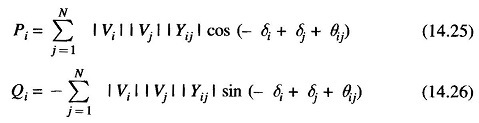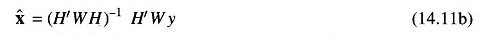## Tracking State Estimation of Power Systems:

Tracking State Estimation of Power Systems is important for real time monitoring of the system. As is well known, the voltages of all real system vary randomly with time and should therefore be considered to be stochastic processes.

It is thus necessary to make use of the sequential estimation techniques in order to obtain the state estimate at any given time point. The power relations in Eqs. (14.25) and (14.26) are still valid but must be rewritten after indicating that the voltage magnitudes and angles are new functions of the discrete time index k.### Some Computational Considerations:

Both the static and the tracking state estimation algorithms presented in the preceding sections are computationally intensive, particularly for large power networks which may have more than 200 important buses. It is, therefore, very important to pay attention to such computational issues as ill conditioning, computer storage and time requirements. However, we need to first consider the question of existence of a solution of the state estimation problem.

### Network Observability:

Consider the static WLSE formula [Eq. (14.11b)] which serves as the starting point for all the algorithms Inverse of information matrix Mnxn=H′ WH should exist otherwise there is no state estimate. This will happen if rank of H is equal to n (no. of state variables). Since one can always choose a non­singular W, so if H has a rank n, the power network is said to be observable.### Problem of I11-conditioning:

Even if the given power system is an observable system in terms of the measurements selected for the state estimation purposes, there is no guarantee that the required inversion of the information matrix will exist. During multiplications of the matrices, there is some small but definite error introduced due to the finite word length and quantization. Whether or not these errors create ill-conditioning of the information matrix may be determined from a knowledge of the condition number of the matrix. This number is defined as the ratio of the largest and the smallest eigen values of the information matrix.

The matrix M becomes more and more ill-conditioned as its condition number increases in magnitude. Some detailed results on power system state estimation using Cholesky factorization techniques. Factorization helps to reduce ill-conditioning but may not reduce the computational burden.

Scroll to Top